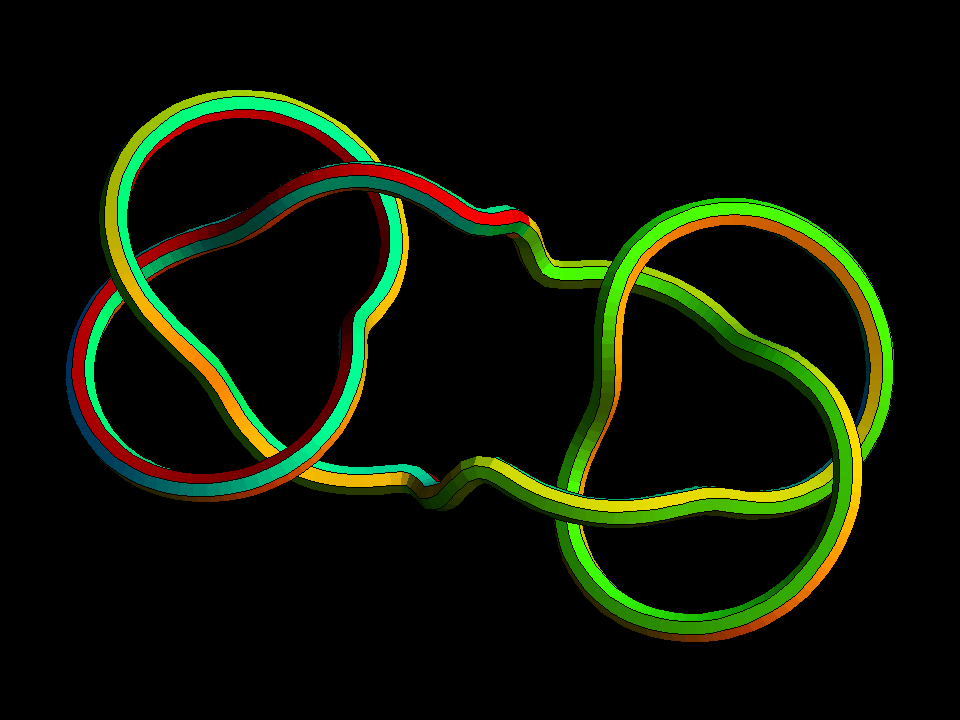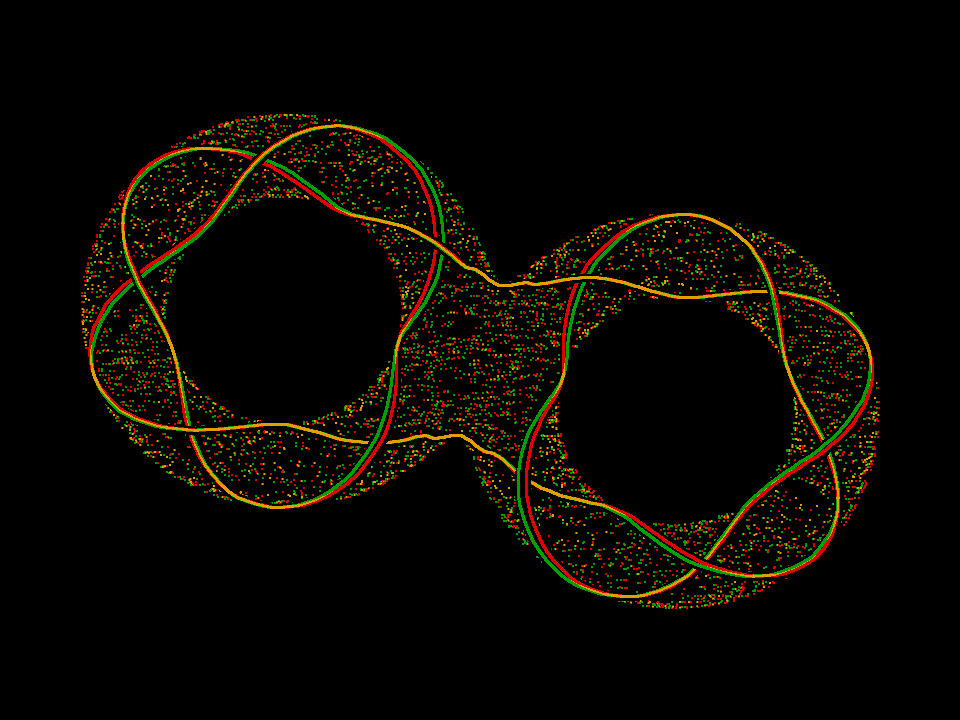# Genus 2 KnotThese knots are “knot sums” of pairs of mirror symmetric torus knots. Every knot can be realized as a knot sum of “prime knots”. Prime knots cannot be realized as sums of simpler knots. The simplest example in this sequence is the Square Knot.These knots are knot sums of pairs of torus knots which can be interchanged by a 180 degree rotation. Notice that the color bands do not always match where beginning and end meet. The tubes are drawn as if they were elastic rods and physics dictates that parallel bands on the straight rod usually do not match when the rod is bent into a closed curve. The simplest example in this sequence is the Granny Knot.These knots are called genus 2 knots, because they can be realized on a surface of genus 2, but not on a simpler surface. Genus 2 surfaces are 2-holed donuts.

Genus_2_Knot.pdf